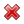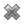# Fight Finance

#### CoursesTagsRandomAllRecentScoresScoreskeithphw $6,011.61 Jade$1,815.80 ZOE HY $860.33 Chu$789.98 royal ne... $750.00 Leehy$713.33 Visitor $650.00 JennyLI$625.61 Visitor $590.00 Visitor$555.33 Visitor $550.00 Visitor$550.00 Visitor $540.00 Visitor$500.00 Yizhou $489.18 Visitor$480.00 Visitor $480.00 Visitor$470.00 Visitor $464.70 Visitor$460.00

Most listed Australian companies pay dividends twice per year, the 'interim' and 'final' dividends, which are roughly 6 months apart.

You are an equities analyst trying to value the company BHP. You decide to use the Dividend Discount Model (DDM) as a starting point, so you study BHP's dividend history and you find that BHP tends to pay the same interim and final dividend each year, and that both grow by the same rate.

You expect BHP will pay a $0.55 interim dividend in six months and a$0.55 final dividend in one year. You expect each to grow by 4% next year and forever, so the interim and final dividends next year will be $0.572 each, and so on in perpetuity. Assume BHP's cost of equity is 8% pa. All rates are quoted as nominal effective rates. The dividends are nominal cash flows and the inflation rate is 2.5% pa. What is the current price of a BHP share? A company announces that it will pay a dividend, as the market expected. The company's shares trade on the stock exchange which is open from 10am in the morning to 4pm in the afternoon each weekday. When would the share price be expected to fall by the amount of the dividend? Ignore taxes. The share price is expected to fall during the: Stocks in the United States usually pay quarterly dividends. For example, the retailer Wal-Mart Stores paid a$0.47 dividend every quarter over the 2013 calendar year and plans to pay a $0.48 dividend every quarter over the 2014 calendar year. Using the dividend discount model and net present value techniques, calculate the stock price of Wal-Mart Stores assuming that: • The time now is the beginning of January 2014. The next dividend of$0.48 will be received in 3 months (end of March 2014), with another 3 quarterly payments of $0.48 after this (end of June, September and December 2014). • The quarterly dividend will increase by 2% every year, but each quarterly dividend over the year will be equal. So each quarterly dividend paid in 2015 will be$0.4896 ($=0.48×(1+0.02)^1$), with the first at the end of March 2015 and the last at the end of December 2015. In 2016 each quarterly dividend will be $0.499392 ($=0.48×(1+0.02)^2$), with the first at the end of March 2016 and the last at the end of December 2016, and so on forever. • The total required return on equity is 6% pa. • The required return and growth rate are given as effective annual rates. • All cash flows and rates are nominal. Inflation is 3% pa. • Dividend payment dates and ex-dividend dates are at the same time. • Remember that there are 4 quarters in a year and 3 months in a quarter. What is the current stock price? Stocks in the United States usually pay quarterly dividends. For example, the software giant Microsoft paid a$0.23 dividend every quarter over the 2013 financial year and plans to pay a $0.28 dividend every quarter over the 2014 financial year. Using the dividend discount model and net present value techniques, calculate the stock price of Microsoft assuming that: • The time now is the beginning of July 2014. The next dividend of$0.28 will be received in 3 months (end of September 2014), with another 3 quarterly payments of $0.28 after this (end of December 2014, March 2015 and June 2015). • The quarterly dividend will increase by 2.5% every year, but each quarterly dividend over the year will be equal. So each quarterly dividend paid in the financial year beginning in September 2015 will be$ 0.287 $(=0.28×(1+0.025)^1)$, with the last at the end of June 2016. In the next financial year beginning in September 2016 each quarterly dividend will be $0.294175 $(=0.28×(1+0.025)^2)$, with the last at the end of June 2017, and so on forever. • The total required return on equity is 6% pa. • The required return and growth rate are given as effective annual rates. • Dividend payment dates and ex-dividend dates are at the same time. • Remember that there are 4 quarters in a year and 3 months in a quarter. What is the current stock price? A share currently worth$100 is expected to pay a constant dividend of $4 for the next 5 years with the first dividend in one year (t=1) and the last in 5 years (t=5). The total required return is 10% pa. What do you expected the share price to be in 5 years, just after the dividend at that time has been paid? You are an equities analyst trying to value the equity of the Australian telecoms company Telstra, with ticker TLS. In Australia, listed companies like Telstra tend to pay dividends every 6 months. The payment around August is called the final dividend and the payment around February is called the interim dividend. Both occur annually. • Today is mid-March 2015. • TLS's last interim dividend of$0.15 was one month ago in mid-February 2015.
• TLS's last final dividend of \$0.15 was seven months ago in mid-August 2014.

Judging by TLS's dividend history and prospects, you estimate that the nominal dividend growth rate will be 1% pa. Assume that TLS's total nominal cost of equity is 6% pa. The dividends are nominal cash flows and the inflation rate is 2.5% pa. All rates are quoted as nominal effective annual rates. Assume that each month is exactly one twelfth (1/12) of a year, so you can ignore the number of days in each month.

Calculate the current TLS share price.